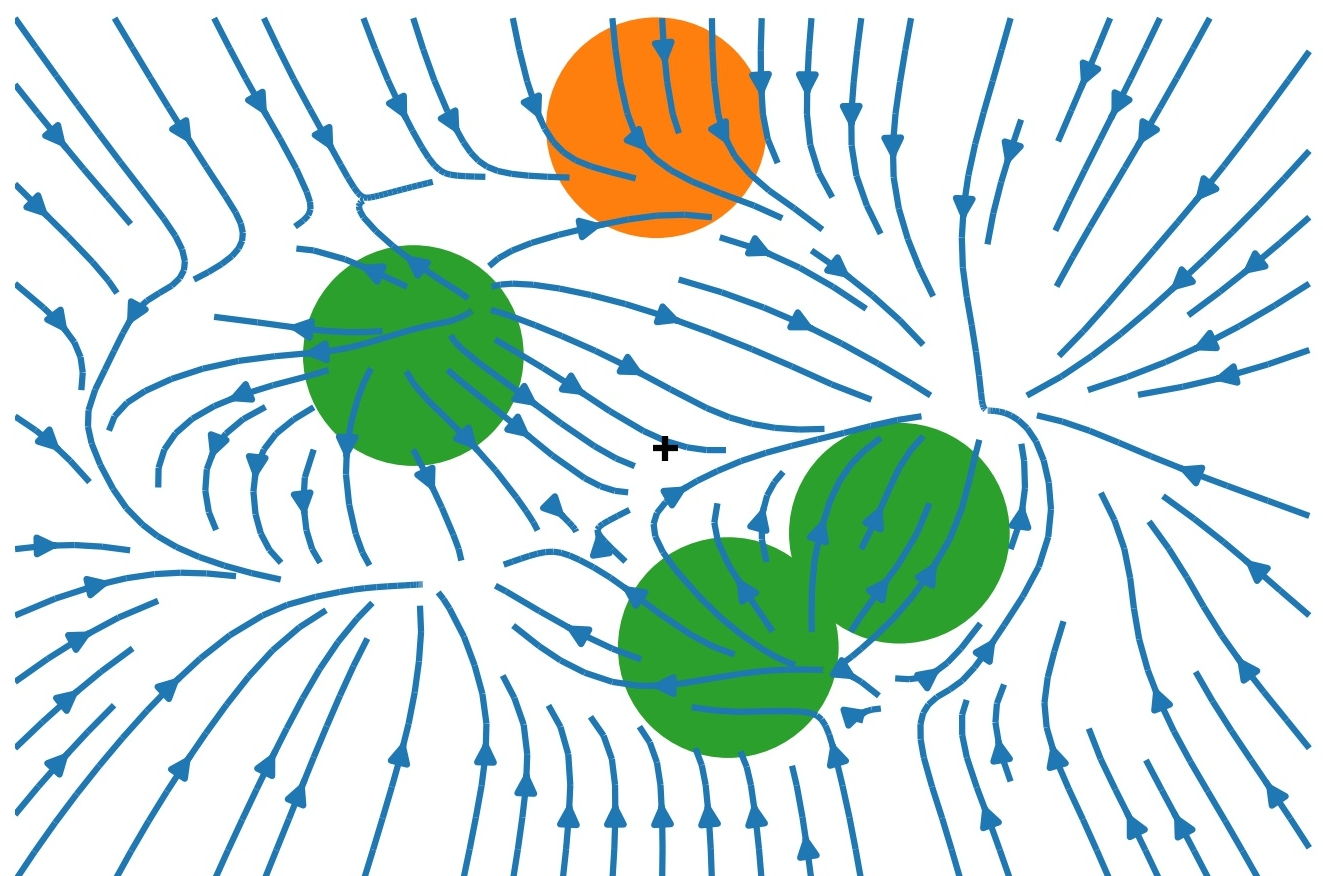# ML and SM 2

### Recap

• Lecture 1 introduced idea of Variational Inference (VI)

• Turns inference in latent variable models into optimization

• Today: how to leverage neural networks & automatic differentiation

• Model of choice: Variational Autoencoder

$$\DeclareMathOperator*{\E}{\mathbb{E}} \newcommand{\cE}{\mathcal{E}} \newcommand{\R}{\mathbb{R}} \newcommand{\bx}{\mathbf{x}} \newcommand{\bz}{\mathbf{z}} \newcommand{\br}{\mathbf{r}} \newcommand{\bv}{\mathbf{v}} \newcommand{\bmu}{\boldsymbol{\mu}} \newcommand{\bSigma}{\boldsymbol{\Sigma}} \newcommand{\bzeta}{\boldsymbol{\zeta}}$$

### VI redux

• Model defined by prior $p(z)$ and generative model $p_\phi(x|z)$

• Similar model for posterior $q_\theta(z|x)$

• Two representatios of $p(x,z)$: forward and backward $$p_\text{F}(x,z)= p_\theta(x|z)p(z),\qquad p_\text{B}(x,z)= q_\phi(z|x)p_\text{D}(x).$$ where $p_\text{D}(x)$ is data distribution

• KL between these two models $$D_\text{KL}(p_\text{B}||p_\text{F})= \E_{x\sim \text{Data}}\left[\E_{z\sim q_\phi(\cdot|x)}\left[\log\left(\frac{q_\phi(z|x)p_\text{D}(x)}{p_\theta(x|z)p(z)}\right)\right]\right]\geq 0.$$ or $$H[p_\text{D}]\leq \E_{x\sim \text{Data}}\left[\E_{z\sim q_\phi(\cdot|x)}\left[\log\left(\frac{q_\phi(z|x)}{p_\theta(x|z)p(z)}\right)\right]\right].$$

• RHS doesn’t involve $p_\text{D}(x)$ explicitly, only expectation. This is implemented as empirical average over (batches of) data

• RHS often presented as

$$\E_{x\sim \text{Data}}\left[D_\text{KL}(q_\phi(\cdot|x)||p)-\E_{z\sim q_\phi(\cdot|x)}\left[\log p_\theta(x|z)\right]\right].$$

• First term small when posterior matches prior

• Second small when model matches data (reconstruction error)

### Variational autoencoder• Autoencoder trained to return outputs close to inputs

• Not trivial if $\text{dim}\,\textbf{h}<\text{dim}\,\textbf{x}$!

• We have a loss function for VI in autoencoder framework $$\mathcal{L}(\theta,\phi)=\E_{x\sim \text{Data}}\left[D_\text{KL}(q_\phi(\cdot|x)||p)-\E_{z\sim q_\phi(\cdot|x)}\left[\log p_\theta(x|z)\right]\right]$$

• We need

• To parameterize $p_\theta(x|z)$ and $q_\phi(z|x)$ using NNs.
• To take gradients of the loss function to perform optimization.
• Let’s look at these in turn.

### Parameterization

• $\bz\in \R^{H}$, $\bx\in \R^{D}$

• For encoder $q_\phi(\bz|\bx)$, choose $\mathcal{N}(\bmu_\phi(\bx),\bSigma_\phi(\bx))$

• If prior is $\mathcal{N}(0,\mathbb{1})$ the KL term loss can be evaluated explicitly.

• $\bmu_\phi(\bx)$ and $\bSigma_\phi(\bx)$ are parameterized using NNs, with architecture adapted to the data e.g. Convolutional neural networks for images

• Similarly for decoder $p_\theta(\cdot|\bz)=\mathcal{N}(\bmu'_\theta(\bz),\bSigma'_\theta(\bz))$

• Second term of loss involves $$-\log p_\theta(\bx|\bz) = \frac{1}{2}(\bx-\bmu'_\theta(\bz))^T\bSigma'^{-1}_\theta(\bz)(\bx-\bmu'_\theta(\bz))+\frac{1}{2}\log\det\bSigma_\theta'(\bz)+\text{const.},$$ encourages mean output $\bmu’_\theta(\bz)$ to be close to $\bx$

• Required expectation over $\bz$ requires Monte Carlo

• Problem: expectation depends on parameters $\phi$, and we want derivatives

• What do we do?

### Reparameterization trick

• If you have $\zeta\sim\mathcal{N}(0,1)$ then $\sigma \zeta +\mu\sim \mathcal{N}(\mu,\sigma^2)$

• Separates parameters from sampling, so that a Monte Carlo estimate of an expectation $$\E_{x\sim \mathcal{N}(\mu,\sigma^2)}\left[f(x)\right]\approx \frac{1}{S}\sum_{s=1}^S f(\sigma z_s + \mu)$$ is explicitly a function of $\sigma$ and $\mu$, so derivatives may be taken

• Generalizes to multivariate Gaussian: $\bz\sim \bSigma_\phi^{1/2}(\bx)\bzeta+\mu_\phi(\bx)$.

### More practicalities

• In practice a single $\bz$ sample is usually found to provide useful gradients for optimization

• Large datasets usually split into batches (sometimes called mini-batches)

• For batch of size $B$ loss function is estimated using $B$ iid $\bzeta_b\sim \mathcal{N}(0,\mathbb{1})$ $$\mathcal{L}(\theta,\phi)\approx\frac{1}{B}\sum_{b=1}^B\left[D_\text{KL}(q_\phi(\cdot|\bx_b)||p)-\log p_\theta(\bx_b|\bSigma_\phi^{1/2}(\bx_b)\bzeta_b+\mu_\phi(\bx_b))\right]$$

• Gradients calculated by automatic differentiation, implemented in all modern DL libraries

• There’s a great deal of craft to the business of training…

### Interpretability

• One promise of latent variable models is an interpretable latent space

• Moving in lower dimensional latent space $\R^H$ allows us to explore the manifold in which the data is embedded in $\R^D$

• Some issues:

1. Loss function doesn’t require that the latent space is used at all. If decoder model $p_\theta(\bx|\bz)$ is rich enough may have $p_\theta(\bx|\bz)\approx p_\text{D}(\bx)$. By Bayes’ theorem posterior is $$\frac{p_\theta(\bx|\bz)p(\bz)}{p_\text{D}(\bx)}\approx p(\bz),$$ same as the prior! This is posterior collapse

2. No guarantee that latent space is used nicely, e.g. with variables for colour, shape, position, etc. (disentangled representation). One problem: prior $\mathcal{N}(0,\mathbb{1})$ is rotationally invariant, so lifting symmetry is necessary.

### Compression with VAEs: bits back

• In Lecture 1 I suggested that good probabilistic models could give better compression

• How does this work for latent variable models like VAE?

• Problem, as always, is that model doesn’t have explicit $p_\text{M}(x)$: marginalizing over latent variables is intractable.

• Recall that loss function of VAE is based

$$H[p_\text{D}]\leq \E_{x\sim \text{Data}}\left[\E_{z\sim q_\phi(\cdot|x)}\left[\log\left(\frac{q_\phi(z|x)}{p_\theta(x|z)p(z)}\right)\right]\right].$$

• Split RHS into three terms

$$\E_{x\sim \text{Data}}\left[\E_{z\sim q_\phi(\cdot|x)}\left[\log\left(q_\phi(z|x)\right)-\log\left(p_\theta(x|z)\right)-\log\left(p(z)\right)\right]\right].$$

• Remember $-\log_2 p(x)$ is length in bits of optimal encoding of $x$. Last two terms could be interpreted as

1. Given data $x$ we sample $z\sim q_\phi(\cdot|x)$.
2. We encode $x$ using the distribution $p_\theta(\cdot|z)$, then
3. Encode $z$ using the prior $p(\cdot)$.
• For decoding, go in reverse

1. Decode $z$ using the prior $p(z)$.
2. Decode $x$ using $p_\theta(\cdot|z)$
• We’ll never reach Shannon bound this way, however, because of the negative first term in

$$\E_{x\sim \text{Data}}\left[\E_{z\sim q_\phi(\cdot|x)}\left[\log\left(q_\phi(z|x)\right)-\log\left(p_\theta(x|z)\right)-\log\left(p(z)\right)\right]\right].$$

• We need to make the code shorter. How?
• Remember that Shannon bound applies in limit of $N\to\infty$ iid data

• Imagine a semi-infinite bit stream mid-way through encoding

• We decode part of already encoded bitstream using $q_\phi(\cdot|x)$

• Result is $z\sim q_\phi(\cdot|x)$: use for encoding $x$ as described above

• These are bits back: remove $H(q_\phi(\cdot|x))$ bits on average

• Allows us to reach the Shannon bound

• When decoding data, the last thing we do for each $x$ is encode $z$ back to the bitstream using $q_\phi(\cdot|x)$

The VAE framework is quite general, and in recent years has been elaborated in various ways.

### Markov chain autoencoders (??)

• Up to now our encoder and decoder were just Gaussian models

• Can we produce a model with a richer distribution?

• Make forward and backward models Markov processes with $T$ steps $$p_\text{F}(z_0,\ldots x=z_T) = p_\theta(x=z_T|z_{T-1})p_\theta(z_{T-1}|z_{T-2})\cdots p_\theta(z_1|z_{0})p(z_0)$$ $$p_\text{B}(z_0,\ldots \ldots x=z_T) = q_\phi(z_0|z_{1})\cdots q_\phi(z_{T-2}|z_{T-1})q_\phi(z_{T-1}|z_T)p_\text{D}(x=z_T)$$

• Loss function is

$$H[p_\text{D}]\leq \E_{z\sim p_\text{B}}\left[\log \left(\frac{q_\phi(z_0|z_1)}{p(z_0)}\right)+\sum_{t=0}^{T-2}\log\left(\frac{q_\phi(z_{t+1}|z_{t+2})}{p_\theta(z_{t+1}|z_t)}\right)\right].$$

• Can pass to continuous time limit, in which case $z_t$ described by stochastic differential equation (SDE). $$dz_t = \mu_\theta(z_t)dt + dW_t$$ $W_t$ is $\R^H$ dimensional Brownian motion, $\mu_\theta(z_t)$ is a parameterized drift

• One forward and one backward SDE

• Model is separate from implementation of dynamics. Solve SDE by whatever method you like: AD through solution.

• Possible applications

1. Infer the trajectories that led to measured outcomes in stochastic dynamics.

• Forward model describes a simulation of a physical system – e.g. molecular dynamics simulation of a biomolecule
• Backward model can be used to infer trajectories that led to some measured states $z_T$.
2. Fix the backward model and just learn the forward model. Seems strange from point of view of finding posterior

### Denoising Diffusion Probabilistic Models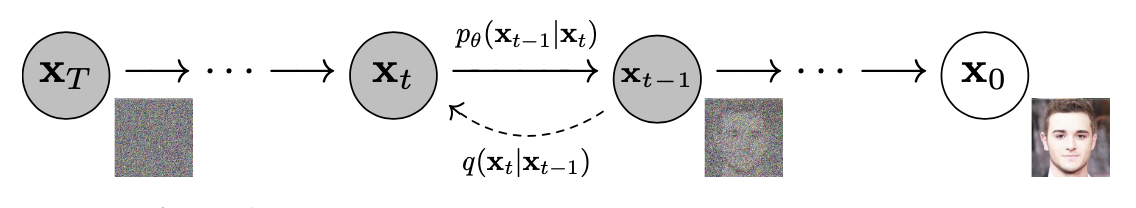### Normalizing flows

• Autoencoders conceived for $H<D$

• By taking $\R^H=\R^D$ can make contact with: Normalizing Flows

• Take $\bSigma_\phi$ and $\bSigma’\theta\to 0$, so that $q\phi(\bz|\bx)$ and $p_\theta(\bx|\bz)$ become deterministic

$$\bz = \mu_\phi(\bx),\qquad \bx = \mu’_\theta(\bz).$$

• $D_\text{KL}\neq 0$ only if they are inverses
• What is KL? $$q_\phi(\cdot|\bx) = \frac{1}{\sqrt{(2\pi)^{D} \det\bSigma_\phi(\bx)}} \exp\left[-\frac{1}{2}(\bz-\bmu_\phi(\bx))^T\bSigma^{-1}_\phi(\bx)(\bz-\bmu_\phi(\bx))\right],$$

• KL involves the ratio $$\frac{q_\phi(\bz|\bx)}{p_\theta(\bx|\bz)}$$

• When $\bz$ and $\bx$ are inverses $$\frac{q_\phi(\bz|\bx)}{p_\theta(\bx|\bz)}\longrightarrow \sqrt{\frac{\det\bSigma'_\theta(\bz)}{\det\bSigma_\phi(\bx)}}=\det \left(\frac{\partial\bx}{\partial\bz}\right).$$

• If $\bz$ described by $p(\bz)$ then $\bx=\mu’_\theta(\bz)$ has density $$\det\left(\frac{\partial\bz}{\partial\bx}\right) p(\mu_\phi(\bx)).$$ i.e. we map to $\bz$ and evaluate density there, accounting for Jacobian

• In deterministic limit, KL becomes $$D_\text{KL}(p_\text{B}||p_\text{F})\longrightarrow -\E_{x\sim \text{Data}}\left[\log\det \left(\frac{\partial\bz}{\partial\bx}\right)+\log p(\mu_\phi(\bx))\right].$$

• Challenge: construct flexible, invertible models with tractable Jacobians (determinant is $O(D^3)$)

• Stack simpler transformations, each invertible with known Jacobian.

### Learning the path integral

Barr, Gispen, Lamacraft (2020)

### Feynman–Kac formula

• For “imaginary time” Schrödinger $$\left[-\frac{\nabla^2}{2m}+V(\br_i)\right]\psi(\br,t) = -\partial_t\psi(\br,t)$$
• Feynman–Kac formula expresses $\psi(\br,t)$ as expectation… $$\psi(\br_2,t_2) = \E_{\br_t}\left[\exp\left(-\int_{t_1}^{t_2}V(\br_t)dt\right)\psi(\br_{t_1},t_1)\right]$$
...over Brownian paths with $\br_{t_{2}}=\br_{2}$
• For $t\to\infty$: $\psi(\br,t)\to e^{-E_0 t}\varphi_0(\br)$
• Path integral Monte Carlo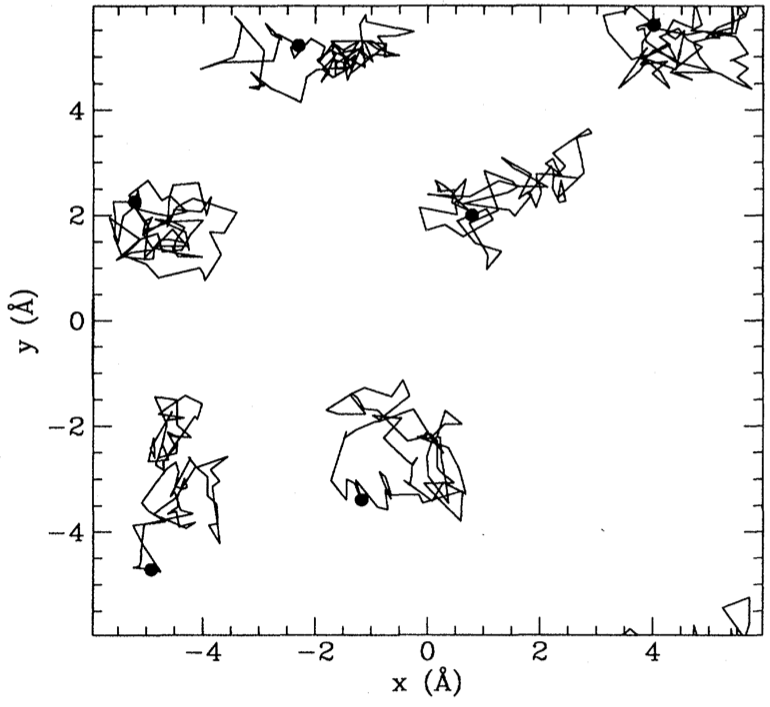Ceperley, RMP (1995)

### Loss function

• FK formula defines path measure $\mathbb{P}_\text{FK}$

• Jamison (1974): process is Markovian $$d\br_t = d\mathbf{W}_t + \bv(\br_t,t)dt$$

• Model drift $\bv(\br,t)$ defines measure $\mathbb{P}_\bv$

• $D_\text{KL}(\mathbb{P}\bv\lvert\rvert \mathbb{P}\text{FK})=\E_{\mathbb{P}\bv}\left[\log\left(\frac{d\mathbb{P}\bv}{d\mathbb{P}_\text{FK}}\right)\right]$ is our loss function

• RL / Optimal Control formulation of QM (Holland, 1977)

### Training

• Relative likelihood (Radon–Nikodym derivative; Girsanov theorem)

$$\log\left(\frac{d\mathbb{P}_{\bv}}{d\mathbb{P}_\text{FK}}\right) =\ell_T - E_0 T+\log\left(\frac{\varphi_0(\br_0)}{\varphi_0(\br_T)}\right)$$ $$\ell_T\equiv \int_0^T \bv(\br_t) \cdot d\mathbf{W}_t+\int_0^T dt\left(\frac{1}{2}|\bv(\br_t)|^2+V(\br_t)\right)$$

• Monte Carlo estimate of $D_\text{KL}(\mathbb{P}\bv\lvert\rvert \mathbb{P}\text{FK})=\E_{\mathbb{P}\bv}\left[\log\left(\frac{d\mathbb{P}\bv}{d\mathbb{P}_\text{FK}}\right)\right]$

• $\br^{(b)}_{t}$ from SDE discretization. Analogous to reparameterization trick

• $D_\text{KL}(\mathbb{P}\bv\lvert\rvert \mathbb{P}\text{FK})\geq 0$ so $\E_{\mathbb{P}_\bv}\left[\ell_T\right]\geq E_0T$

• Suggests strategy:

1. Represent $\bv_\theta(\br) = \textsf{NN}_\theta(\br)$
2. Integrate batch of SDE trajectories
3. Backprop through the (MC estimated) cost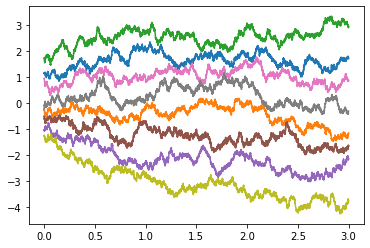### Hydrogen Molecule

$$H = -\frac{\nabla_1^2+\nabla_2^2}{2}+ \frac{1}{|\br_1-\br_2|}- \sum_{i=1,2}\left[\frac{1}{|\br_i-\hat{\mathbf{z}} R/2|} + \frac{1}{|\br_i+\hat{\mathbf{z}}R/2|}\right]$$

• Equilibrium proton separation $R=1.401$, $E_0= -1.174476$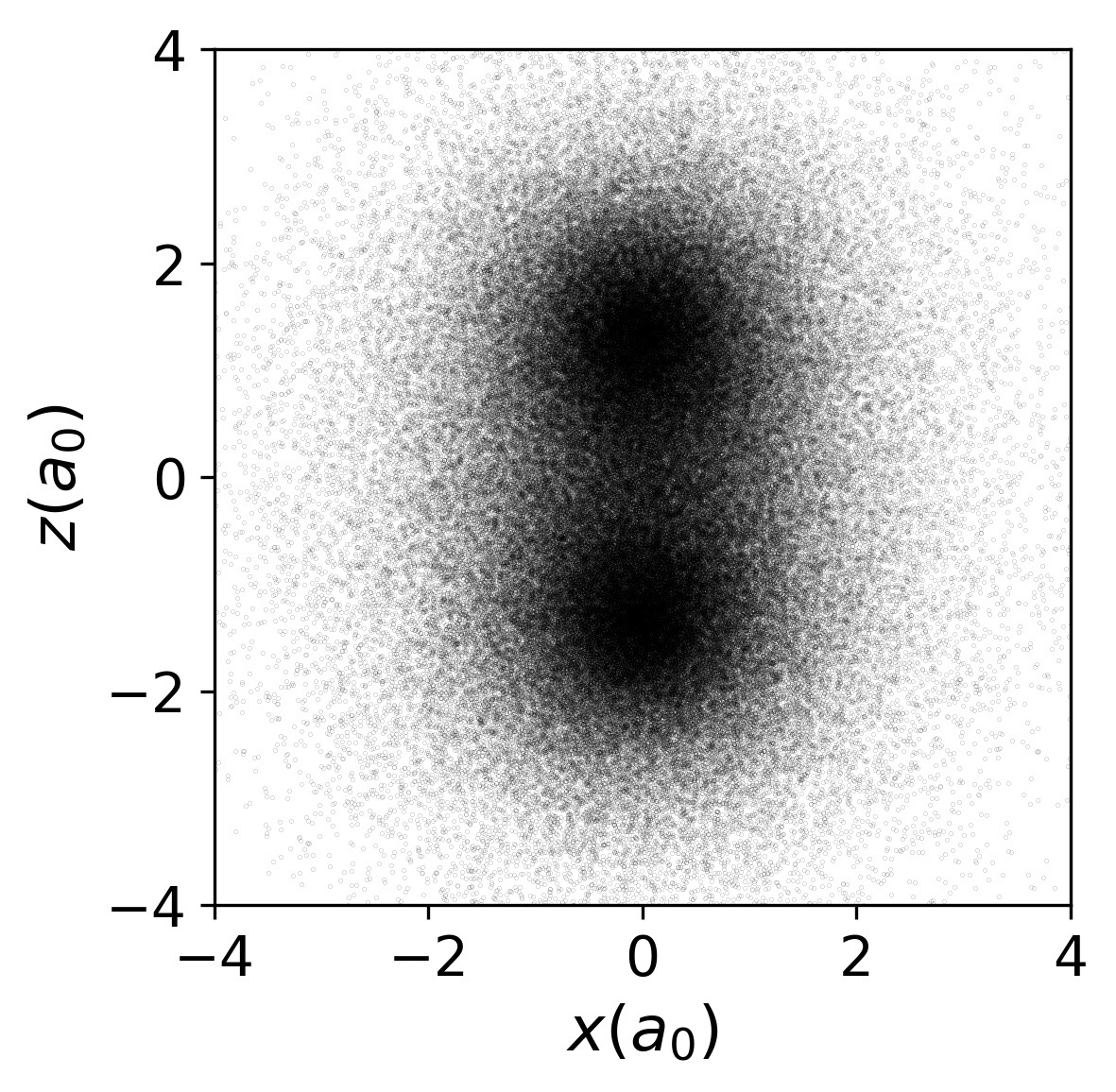### 2D Gaussian Bosons

\begin{align} H&=\frac{1}{2}\sum_i \left[-\nabla_i^2 +\br_i^2\right]+\sum_{i<j}U(\br_i-\br_j)\\ U(\br) &=\frac{g}{\pi s^2}e^{-\br^2/s^2} \end{align}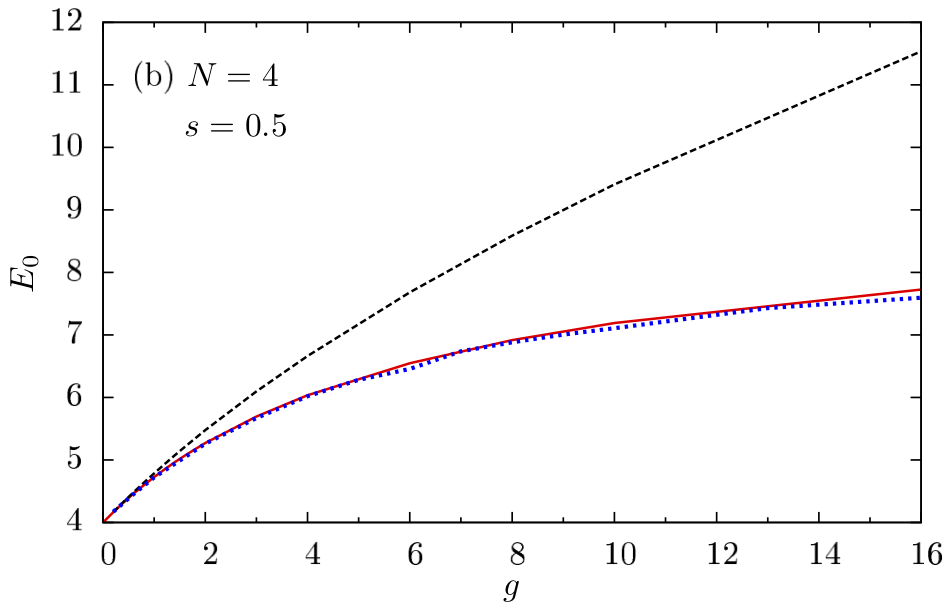• Drift Visualization ($g=15$, $s=1/2$)